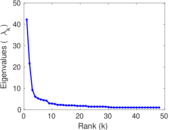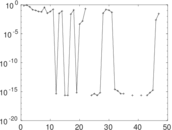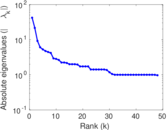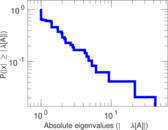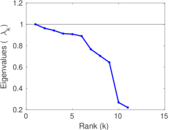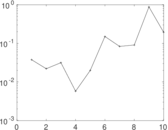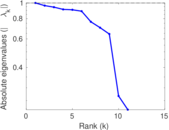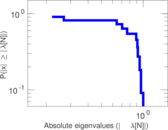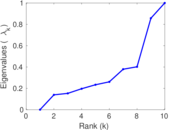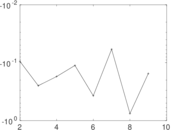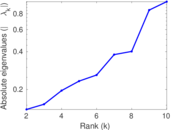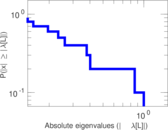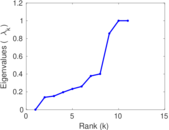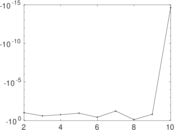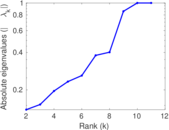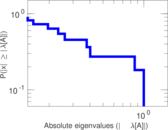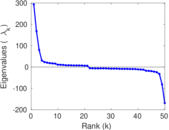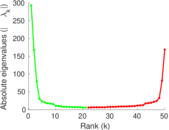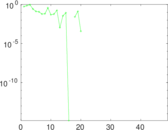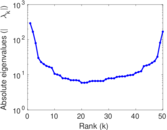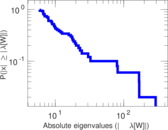# Wikibooks edits (na)

This is the bipartite edit network of the Nauru Wikibooks. It contains users and pages from the Nauru Wikibooks, connected by edit events. Each edge represents an edit. The dataset includes the timestamp of each edit.

 Code `bna` Internal name `edit-nawikibooks` Name Wikibooks edits (na) Data source http://dumps.wikimedia.org/ AvailabilityDataset is available for download Consistency checkDataset passed all tests Category Authorship network Dataset timestamp 2017-10-20 Node meaning User, article Edge meaning Edit Network formatBipartite, undirected Edge typeUnweighted, multiple edges Temporal dataEdges are annotated with timestamps

## Statistics

 Size n = 300 Left size n1 = 50 Right size n2 = 250 Volume m = 414 Unique edge count m̿ = 280 Wedge count s = 5,918 Claw count z = 141,152 Cross count x = 2,566,855 Square count q = 27 4-Tour count T4 = 24,764 Maximum degree dmax = 136 Maximum left degree d1max = 136 Maximum right degree d2max = 42 Average degree d = 2.760 00 Average left degree d1 = 8.280 00 Average right degree d2 = 1.656 00 Fill p = 0.022 400 0 Average edge multiplicity m̃ = 1.478 57 Size of LCC N = 101 Diameter δ = 6 50-Percentile effective diameter δ0.5 = 1.719 68 90-Percentile effective diameter δ0.9 = 3.570 07 Median distance δM = 2 Mean distance δm = 2.507 68 Gini coefficient G = 0.630 010 Balanced inequality ratio P = 0.251 208 Left balanced inequality ratio P1 = 0.185 990 Right balanced inequality ratio P2 = 0.374 396 Relative edge distribution entropy Her = 0.836 591 Power law exponent γ = 5.978 79 Tail power law exponent γt = 2.811 00 Tail power law exponent with p γ3 = 2.811 00 p-value p = 0.273 000 Left tail power law exponent with p γ3,1 = 2.011 00 Left p-value p1 = 0.259 000 Right tail power law exponent with p γ3,2 = 3.751 00 Right p-value p2 = 0.033 000 0 Degree assortativity ρ = −0.226 078 Degree assortativity p-value pρ = 0.000 135 854 Spectral norm α = 42.201 9 Algebraic connectivity a = 0.138 746 Spectral separation |λ1[A] / λ2[A]| = 1.952 56 Controllability C = 200 Relative controllability Cr = 0.666 667

## Plots

### Fruchterman–Reingold graph drawing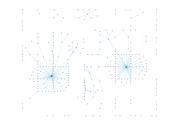### Degree distribution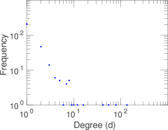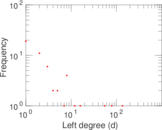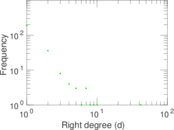### Cumulative degree distribution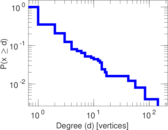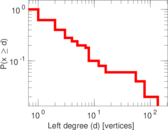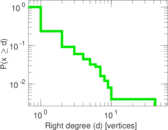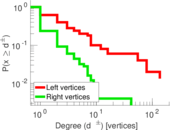### Lorenz curve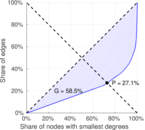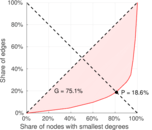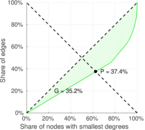### Spectral distribution of the adjacency matrix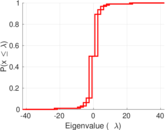### Spectral distribution of the normalized adjacency matrix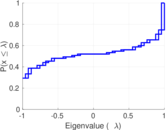### Spectral distribution of the Laplacian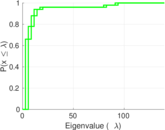### Spectral graph drawing based on the adjacency matrix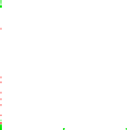### Spectral graph drawing based on the Laplacian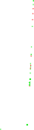### Spectral graph drawing based on the normalized adjacency matrix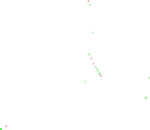### Degree assortativity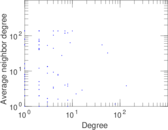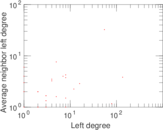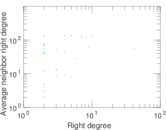### Zipf plot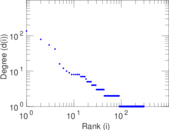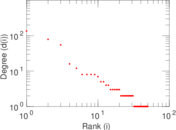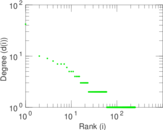### Hop distribution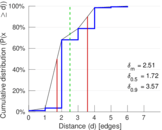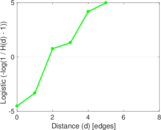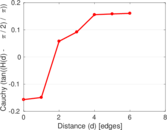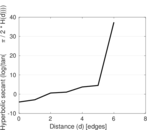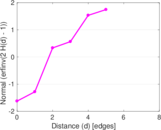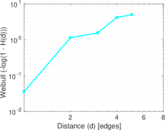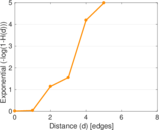### Double Laplacian graph drawing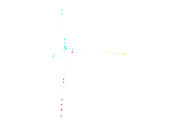### Delaunay graph drawing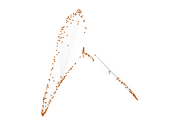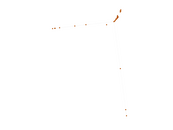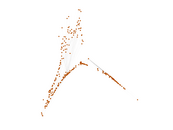### Edge weight/multiplicity distribution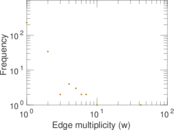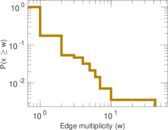### Temporal distribution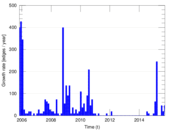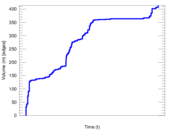### Temporal hop distribution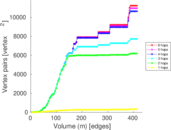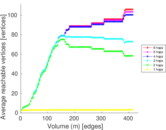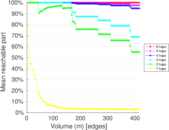### Diameter/density evolution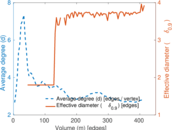### Matrix decompositions plots